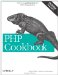# Recipe 3.8. Validating a Date

#### 3.8.1. Problem

You want to check if a date is valid. For example, you want to make sure a user hasn't provided a birthdate such as February 30, 1962.

#### 3.8.2. Solution

Use checkdate( ):

`\$valid = checkdate(\$month,\$day,\$year);`

#### 3.8.3. Discussion

The function checkdate( ) returns TRue if \$month is between 1 and 12, \$year is between 1 and 32767, and \$day is between 1 and the correct maximum number of days for \$month and \$year. Leap years are correctly handled by checkdate( ), and dates are rendered using the Gregorian calendar.

Because checkdate( ) has such a broad range of valid years, you should do additional validation on user input if, for example, you're expecting a valid birthdate. The longest confirmed human lifespan is 122 years old. To check that a birthdate indicates that a user is between 18 and 122 years old, use the pc_checkbirthdate( ) function shown in Example 3-19.

##### pc_checkbirthdate( )

 ` \$min_year) && (\$year < \$max_year)) { return true; } elseif ((\$year == \$max_year) && ((\$month < \$this_month) || ((\$month == \$this_month) && (\$day <= \$this_day)))) { return true; } elseif ((\$year == \$min_year) && ((\$month > \$this_month) || ((\$month == \$this_month && (\$day > \$this_day))))) { return true; } else { return false; } } // check December 3, 1974 if (pc_checkbirthdate(12,3,1974)) { print "You may use this web site."; } else { print "You are too young to proceed."; exit(); } ?>`

The function first uses checkdate( ) to make sure that \$month, \$day, and \$year represent a valid date. Various comparisons then make sure that the supplied date is in the range set by \$min_age and \$max_age.

If \$year is noninclusively between \$min_year and \$max_year, the date is definitely within the range, and the function returns true. If not, some additional checks are required. If \$year equals \$max_year (e.g., in 2002, \$year is 1984), \$month must be before the current month. If \$month equals the current month, \$day must be before or equal to the current day. If \$year equals \$min_year (e.g., in 2002, \$year is 1880), \$month must be after the current month. If \$month equals the current month, \$day must be after the current day. If none of these conditions are met, the supplied date is outside the appropriate range, and the function returns false.

The function returns true if the supplied date is exactly \$min_age years before the current date, but false if the supplied date is exactly \$max_age years after the current date. That is, it would let you through on your 18th birthday, but not on your 123rd.

Documentation on checkdate( ) at http://www.php.net/checkdate; information about Jeanne Calment, the person with the longest confirmed lifespan, is at http://en.wikipedia.org/wiki/Jeanne_Calment .PHP Cookbook: Solutions and Examples for PHP Programmers
ISBN: 0596101015
EAN: 2147483647
Year: 2006
Pages: 445

Similar book on Amazon